Next: Voltage-Controlled Voltage Sources Up: 3.1 Free Energy of Previous: 3.1.1 Notation

## 3.1.2 Electrostatic Energy

Using the  capacitance matrix, charges and potentials are related by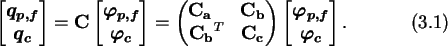The sub-matrices Ca, Cb, and Cc have dimensions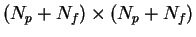,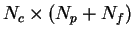, and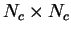respectively. The capacitance matrix is a symmetric matrix, which allows efficient storage and handling. Consequently Ca and Cc are symmetric. The unknown quantities of charges on potential-nodes and floating-nodes and potentials of charge-nodes,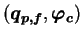, can be written in terms of the known quantities which are the potentials on potential-nodes and floating-nodes and the charges on charge-nodes.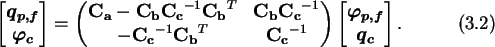The potentials of the floating-nodes must be derived from the equations of the macro-nodes, which are the known sum of charge of each macro-node, and the individual potential differences given by the voltage sources comprising a macro-node. An example of a macro-node is shown in Fig. 3.2.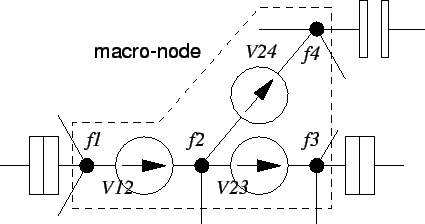The voltage sources give a set of Nfm-1 equations, where Nfm is the number of nodes in a macro-node, which are for the example of Fig. 3.2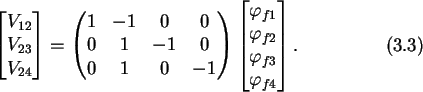Voltage sources must not be  short circuited. That is, no loop consisting solely of voltage sources may exist in the circuit. With the additional equation for the charge of the macro-node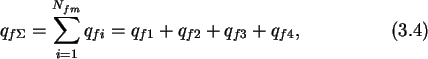which is taken from (3.2) by adding all Nfm lines for the node charges comprising one macro-node, one has Nfm equations for the potentials of the Nfm floating-nodes.

The electrostatic energy of a circuit can be expressed using the relation which links known charges and potentials with the unknown quantities, (3.2), as, (see also (2.5))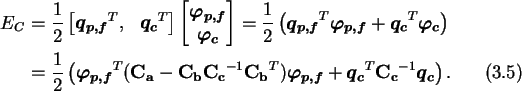Next: Voltage-Controlled Voltage Sources Up: 3.1 Free Energy of Previous: 3.1.1 Notation

Christoph Wasshuber